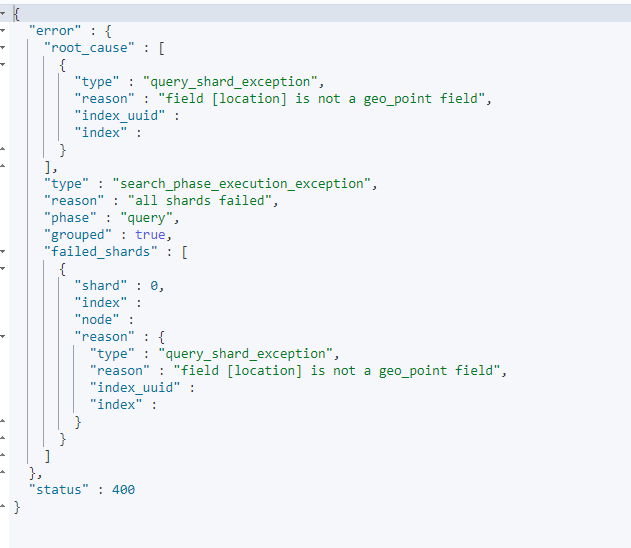There are circumstances when we have a set of coordinates and we want to perform a  geo search on them. More specifically we want to find out if the coordinate used in our search falls within a shape. In that case we can use the Elastic Search geo shape query to achieve that. In this post I’m going to use geo shape query to perform a search and explain how it works.

## What is Geo Shape Query?

Geo Shape query is a type of query we use to see if a coordinate falls within a specific geometric shape. Let’s show this with an example, consider the blow image.

Imagine we already have a coordinate in our database that represent a place which here is represented by a red marker. Now we want to specify a shape on the map (which in this case is a pentagon) to see the our coordinate falls within this shape. If the coordinate falls within this shape then we have a match. Also worth noting that the shape does not have to be a pentagon. But it can be any type of polygon, here’s the definition of polygon.

A plane figure with at least three straight sides and angles, and typically five or more.

So by that definition, this shape is also acceptable.

If you need a set of polygon for testing purposes, I already created one in this link. This tool in google map is also can be a powerful tool to help us to debug our geo shape queries.

## How the Geo Shape Query Works

First we need to create the necessary mappings. In the code below we create a mapping for field `location `with the type `geo_shape`. After that we create some coordinate points.

Now we can run our queries against the created point. Below you see two queries with different shapes. One polygon in the shape of polygon and another in the shape of a triangle. The coordinates use here can visualized in this custom map which I created previously.

Also it’s a good idea to receive `GeoJson` for our search coordinates. This format allows us to work with the data in a programmatic way as opposed to other format which are textual and need string interpretation.

## Geo Shape Query Necessary Mapping

The type of mapping for geo shape query needs to be `geo_shape`. But it can also work with type geo-point, on the condition that we use `"relation": "intersect"` instead of `"relation": "within"`. So the following error is only occur when we want to use relation within.

So as an example, we we try to use the following mapping for our geo shape query:

When we try to run a geo shape query, we’re going to receive the following error.

The mapping incompatibility is also applies to other types of query such as `geo-distance`, if we trying to run the following query on a field with geo shape mapping:

This is the error that we’re going to receive.In the next section we’re going to see what type of coordinates we need to send to elastic search.

## What is a Valid Polygon (closed polygon)

In order for the geo shape to work, we need to receive a valid polygon. A valid polygon in this case is a closed polygon.

A polygon is open when the segments do not all connect at the beginning and end. That is, if we draw the polygon starting at one point, we finish drawing at a different point. This is an example of an open polygonal line:A polygon is closed when the segments do connect at the beginning and end. That is, you start to draw the line at a point and finish at the same point. This is an example of a closed polygon:

Worth mentioning that there is an option called coerce that can coerce a polygon  to a closed one. This option suppose to close the polygon for us it an unclosed polygon is passed in. But after I test `"coerce": true` does not work and we still receive the same error.

Here’s how this will be reflected in the coordinates we receive. In the example below, we see that first and last coordinates are repeated exactly to constitute a closed polygon.

Closing a polygon is not always as simple as repeating the first coordinate to the last, so we need to make clear the coordinates we receive are creating a closed polygon. Otherwise the query does not going to work and elastic search is going to return the following error.

## Geo Shape Query Sample

I also created a sample for experiment. The request body for geo shape query can be something like below.

## Geo Shape Query the Other Way Around

So far we had one coordinate, and in our query, we provided an array of coordinates which constitute a shape. Then we looked to see if our target coordinates fell within that shape.

But we can do this also the other way around, that is, we can submit a set of coordinates which constitute a shape. Then we can look if one target coordinate fall within that shape. Let me illustrate what I mean by an example.

Here we have a shape in our index and we search in that shape to see if a coordinate is contained inside that shape.